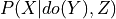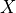# Causal Inference¶

class pgmpy.inference.CausalInference.CausalInference(model, set_nodes=None)[source]

This is an inference class for performing Causal Inference over Bayesian Networks or Structural Equation Models.

This class will accept queries of the form: P(Y | do(X)) and utilize its methods to provide an estimand which:

Parameters:
• model (CausalGraph) – The model that we’ll perform inference over.

• set_nodes (list[node:str] or None) – A list (or set/tuple) of nodes in the Bayesian Network which have been set to a specific value per the do-operator.

Examples

Create a small Bayesian Network. >>> from pgmpy.models import BayesianNetwork >>> game = BayesianNetwork([(‘X’, ‘A’), … (‘A’, ‘Y’), … (‘A’, ‘B’)])

Load the graph into the CausalInference object to make causal queries. >>> from pgmpy.inference.CausalInference import CausalInference >>> inference = CausalInference(game) >>> inference.get_all_backdoor_adjustment_sets(X=”X”, Y=”Y”) >>> inference.get_all_frontdoor_adjustment_sets(X=”X”, Y=”Y”)

References

‘Causality: Models, Reasoning, and Inference’ - Judea Pearl (2000)

Many thanks to @ijmbarr for their implementation of Causal Graphical models available. It served as an invaluable reference. Available on GitHub: https://github.com/ijmbarr/causalgraphicalmodels

estimate_ate(X, Y, data, estimand_strategy='smallest', estimator_type='linear', **kwargs)[source]

Estimate the average treatment effect (ATE) of X on Y.

Parameters:
• X (str) – Intervention Variable

• Y (str) – Target Variable

• data (pandas.DataFrame) – All observed data for this Bayesian Network.

• estimand_strategy (str or frozenset) –

Either specify a specific backdoor adjustment set or a strategy. The available options are:

smallest:

Use the smallest estimand of observed variables

all:

Estimate the ATE from each identified estimand

• estimator_type (str) –

The type of model to be used to estimate the ATE. All of the linear regression classes in statsmodels are available including:

• GLS: generalized least squares for arbitrary covariance

• OLS: ordinary least square of i.i.d. errors

• WLS: weighted least squares for heteroskedastic error

Specify them with their acronym (e.g. “OLS”) or simple “linear” as an alias for OLS.

• **kwargs (dict) –

Keyward arguments specific to the selected estimator. linear:

missing: str

Available options are “none”, “drop”, or “raise”

Returns:

The average treatment effect

Return type:

float

Examples

```>>> import pandas as pd
>>> game1 = BayesianNetwork([('X', 'A'),
...                          ('A', 'Y'),
...                          ('A', 'B')])
>>> data = pd.DataFrame(np.random.randint(2, size=(1000, 4)), columns=['X', 'A', 'B', 'Y'])
>>> inference = CausalInference(model=game1)
>>> inference.estimate_ate("X", "Y", data=data, estimator_type="linear")
```

Returns a list of all adjustment sets per the back-door criterion.

A set of variables Z satisfies the back-door criterion relative to an ordered pair of variabies (Xi, Xj) in a DAG G if:
1. no node in Z is a descendant of Xi; and

2. Z blocks every path between Xi and Xj that contains an arrow into Xi.

Todo

• Backdoors are great, but the most general things we could implement would be Ilya Shpitser’s ID and IDC algorithms. See [his Ph.D. thesis for a full explanation] (https://ftp.cs.ucla.edu/pub/stat_ser/shpitser-thesis.pdf). After doing a little reading it is clear that we do not need to immediatly implement this. However, in order for us to truly account for unobserved variables, we will need not only these algorithms, but a more general implementation of a DAG. Most DAGs do not allow for bidirected edges, but it is an important piece of notation which Pearl and Shpitser use to denote graphs with latent variables.

Parameters:

X (str) – Intervention Variable

Returns:

• frozenset (A frozenset of frozensets)

• Y (str) – Target Variable

Examples

```>>> game1 = BayesianNetwork([('X', 'A'),
...                          ('A', 'Y'),
...                          ('A', 'B')])
>>> inference = CausalInference(game1)
frozenset()
```

References

“Causality: Models, Reasoning, and Inference”, Judea Pearl (2000). p.79.

Identify possible sets of variables, Z, which satisify the front-door criterion relative to given X and Y.

Z satisifies the front-door critierion if:
1. Z intercepts all directed paths from X to Y

2. there is no backdoor path from X to Z

3. all back-door paths from Z to Y are blocked by X

Returns:

frozenset

Return type:

a frozenset of frozensets

References

Causality: Models, Reasoning, and Inference, Judea Pearl (2000). p.82.

get_distribution()[source]

Returns a string representing the factorized distribution implied by the CGM.

Method to test whether adjustment_set is a valid adjustment set for identifying the causal effect of X on Y.

Parameters:
• X (str (variable name)) – The cause/exposure variables.

• Y (str (variable name)) – The outcome variable

Returns:

Minimal adjustment set – A set of variables which are the minimal possible adjustment set. If None, no adjutment set is possible.

Return type:

set or None

Examples

References

 Perkovic, Emilija, et al. “Complete graphical characterization and construction of adjustment sets in Markov equivalence classes of ancestral graphs.” The Journal of Machine Learning Research 18.1 (2017): 8132-8193.

get_proper_backdoor_graph(X, Y, inplace=False)[source]

Returns a proper backdoor graph for the exposure X and outcome Y. A proper backdoor graph is a graph which remove the first edge of every proper causal path from X to Y.

Parameters:
• X (list (array-like)) – A list of exposure variables.

• Y (list (array-like)) – A list of outcome variables

• inplace (boolean) – If inplace is True, modifies the object itself. Otherwise retuns a modified copy of self.

Examples

```>>> from pgmpy.models import BayesianNetwork
>>> from pgmpy.inference import CausalInference
>>> model = BayesianNetwork([("x1", "y1"), ("x1", "z1"), ("z1", "z2"),
...                        ("z2", "x2"), ("y2", "z2")])
>>> c_infer = CausalInference(model)
>>> c_infer.get_proper_backdoor_graph(X=["x1", "x2"], Y=["y1", "y2"])
```

References

 Perkovic, Emilija, et al. “Complete graphical characterization and construction of adjustment sets in Markov equivalence classes of ancestral graphs.” The Journal of Machine Learning Research 18.1 (2017): 8132-8193.

Method to test whether adjustment_set is a valid adjustment set for identifying the causal effect of X on Y.

Parameters:
• X (list (array-like)) – The set of cause variables.

• Y (list (array-like)) – The set of predictor variables.

• adjustment_set (list (array-like)) – The set of variables for which to test whether they satisfy the adjustment set criteria.

Returns:

Is valid adjustment set – Returns True if adjustment_set is a valid adjustment set for identifying the effect of X on Y. Else returns False.

Return type:

bool

Examples

```>>> from pgmpy.models import BayesianNetwork
>>> from pgmpy.inference import CausalInference
>>> model = BayesianNetwork([("x1", "y1"), ("x1", "z1"), ("z1", "z2"),
...                        ("z2", "x2"), ("y2", "z2")])
>>> c_infer = CausalInference(model)
True
```

References

 Perkovic, Emilija, et al. “Complete graphical characterization and construction of adjustment sets in Markov equivalence classes of ancestral graphs.” The Journal of Machine Learning Research 18.1 (2017): 8132-8193.

Test whether Z is a valid backdoor adjustment set for estimating the causal impact of X on Y.

Parameters:
• X (str) – Intervention Variable

• Y (str) – Target Variable

• Z (str or set[str]) – Adjustment variables

Returns:

Is a valid backdoor adjustment set – True if Z is a valid backdoor adjustment set else False

Return type:

bool

Examples

```>>> game1 = BayesianNetwork([('X', 'A'),
...                          ('A', 'Y'),
...                          ('A', 'B')])
>>> inference = CausalInference(game1)
True
```

Test whether Z is a valid frontdoor adjustment set for estimating the causal impact of X on Y via the frontdoor adjustment formula.

Parameters:
• X (str) – Intervention Variable

• Y (str) – Target Variable

• Z (set) – Adjustment variables

Returns:

Is valid frontdoor adjusment – True if Z is a valid frontdoor adjustment set.

Return type:

bool

query(variables, do=None, evidence=None, adjustment_set=None, inference_algo='ve', show_progress=True, **kwargs)[source]

Performs a query on the model of the formwhereis variables,is do and Z is the evidence.

Parameters:
• variables (list) – list of variables in the query i.e. X in.

• do (dict (default: None)) – Dictionary of the form {variable_name: variable_state} representing the variables on which to apply the do operation i.e. Y in.

• evidence (dict (default: None)) – Dictionary of the form {variable_name: variable_state} repesenting the conditional variables in the query i.e. Z in.

• adjustment_set (str or list (default=None)) – Specifies the adjustment set to use. If None, uses the parents of the do variables as the adjustment set.

• inference_algo (str or pgmpy.inference.Inference instance) – The inference algorithm to use to compute the probability values. String options are: 1) ve: Variable Elimination 2) bp: Belief Propagation.

• kwargs (Any) – Additional paramters which needs to be passed to inference algorithms. Please refer to the pgmpy.inference.Inference for details.

Returns:

Queried distribution – A factor object representing the joint distribution over the variables in variables.

Return type:

pgmpy.factor.discrete.DiscreteFactor

Examples

```>>> from pgmpy.utils import get_example_model
>>> model = get_example_model('alarm')
>>> infer = CausalInference(model)
>>> infer.query(['HISTORY'], do={'CVP': 'LOW'}, evidence={'HR': 'LOW'})
<DiscreteFactor representing phi(HISTORY:2) at 0x7f4e0874c2e0>
```